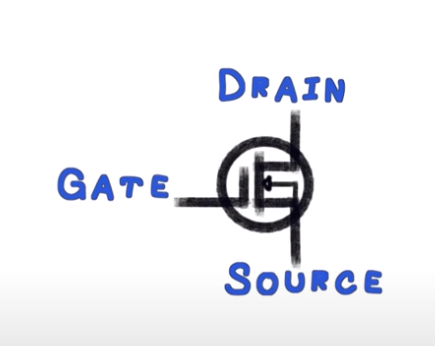##### Page tree
Go to start of banner

# Overview

Metal Oxide Semiconductor Field Effect Transistor

"variable resistors controlled by voltage"# How they Work

MOSFETs work like BJT Transistors but trigger based on voltage instead of current.When voltage is applied between Gate and Source, current is allowed to flow between DRAIN and SOURCE.

MOSFETs are variable resistors controlled by voltage. Depending on the voltage applied at the GATE, the resistance between the DRAIN and SOURCE will vary (known as RDSon).

# Do I Need a Heat Sink?

Calculate the power you are going to need to dissipate.

Where I is the current your application is going to pull.

Let's assume our little motor will pull 1A and RDS=0.035Omhs I

P=R x I2

=RDS x I2

= 0.035 * 12

= 35 mWatts

The formula to compute the amount of power our MOSFET can dissipate without a heat sink is as follows:

PD = ( (MAX(TJ) - (TA) )/ R0JA

We will need a few more items from the data sheet.

MAX(TJ) - Maximum Junction Temperature

TA - Ambiant Temperature (25C)

R0JA - Thermal Resistance, Junction-to-Ambient, Max

How many watts can we dissipate without a heat sink?

PD = ( (MAX(TJ) - (TA) )/ R0JA

=  (175C - 25C )/ 62.5

= 2.4 Watts

NOTE:

The spec sheet will refer to a PD  which is higher than our calculation. This is because it assumes that you can keep the MOSFET at 25C while operating which would mean you would need a heatsink.

# Reference

ReferenceURL
MOSFET Transistorhttps://www.electronics-tutorials.ws/transistor/tran_6.html